Method for Solving Particular Solution of Linear Second Order Ordinary Differential Equations | OMICS International
Journal of Applied & Computational Mathematics
All submissions of the EM system will be redirected to Online Manuscript Submission System. Authors are requested to submit articles directly to Online Manuscript Submission System of respective journal.

# Method for Solving Particular Solution of Linear Second Order Ordinary Differential Equations

Arficho D*

Department of mathematics, Aksum University, Aksum, Ethiopia

*Corresponding Author:
Arficho D
Department of mathematics
Aksum University, Aksum, Ethiopia
Tel: 347753645
E-mail: [email protected]

Received Date: March 10, 2015; Accepted Date: March 25, 2015; Published Date: April 10, 2015

Citation: Arficho D (2015) Method for Solving Particular Solution of Linear Second Order Ordinary Differential Equations. J Appl Computat Math 4:210. doi: 10.4172/2168-9679.1000210

Copyright: © 2015 Arficho D. This is an open-access article distributed under the terms of the Creative Commons Attribution License, which permits unrestricted use, distribution, and reproduction in any medium, provided the original author and source are credited.

Visit for more related articles at Journal of Applied & Computational Mathematics

#### Abstract

In this paper, we derive new method for solving particular solution of linear second order ordinary differential equations whenever one solution of their associated homogeneous differential equations is given. Also, we construct second solution of the associated homogeneous differential equation from this new method. Moreover, we have general solution method of linear second order ordinary differential equations without applying the two famous methods, undetermined coefficients method and variation of parameters method, for solving their particular solution

#### Keywords

Reduction of order; Fundamental set

#### Introduction

A differential equation is an equation that relates an unknown function and one or more of its derivatives of with respect to one or more independent variables [1,2]. If the unknown function depends only on a single independent variable, such a differential equation is ordinary. The order of an ordinary differential equation is the order of the highest derivative that appears in the equation . In real world, there are physical problems that are ordinary differential equations. Thus, we need solution methods to solve these problems. A solution of a differential equation in the unknown function y and the independent variable x on the interval I is a function y(x) that satisfies the differential equation identical for all x in I . A solution of a differential equation with arbitrary parameters is called a general solution. A solution of a differential equation that is free of arbitrary parameters is called a particular solution . A solution in which the dependent variable is expressed solely in terms of the independent variable and constants is said to be an explicit solution. A relation G(x,y) is said to be an implicit solution of an ordinary differential equation on an interval I, provided there exists at least one function f that satisfies the relation as well as the DE on I . Moreover, solutions of differential equations are classified as trivial and non-trivial solutions, general and particular solutions and explicit and implicit solutions. The general solution of linear second order ordinary differential equations consists of solutions of their associated homogeneous differential equations and their particular solutions. There are two famous methods, undetermined coefficients method and variation of parameters method, for solving particular solutions of linear second order ordinary differential equations. Finally, one can solve for particular solution of linear second order ordinary differential equations without applying undetermined coefficients method and variation of parameters method.

#### Linear Higher Order Differential Equations

A differential equation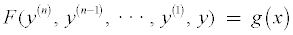(1.1)

is said to be linear if F is a linear function of the variables y(n), y(n−1), · · · , y(1), y . An nth-order linear differential equation in a dependent variable y and independent variable x defined on an interval I Ì R has the form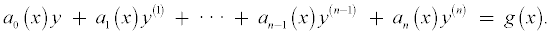(1.2)

If g(x) = 0 for all x in I, then the differential equation is homogeneous, otherwise, it is non-homogeneous .

Linear first order differential equations

The linear first order ordinary differential equation with unknown dependent variable y and independent variable x is defined by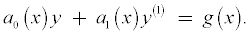(2.1)

Solution of linear first order differential equations: The general solution of the equation in equation 2.1 is given by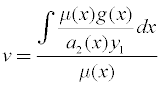(2.2)

where μ(X)=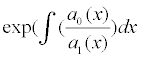Fundamental set of solutions

A set of functions y1(x), y2(x), · · · , fn(x) is said to be linearly dependent on an interval I if there exist constants c1, c2, , cn not all zero, such that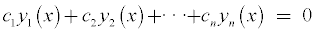for every x in the interval. If the set of functions is not linearly dependent on the interval, it is said to be linearly independent. Any set y1(x), y2(x), · · · , fn(x) of n linearly independent solutions of the homogeneous linear nth-order differential equation on an interval I is said to be a fundamental set of solutions on the interval.

General solution of linear higher order differential equations

The associated homogeneous differential equation of nonhomogeneous linear nth order differential equation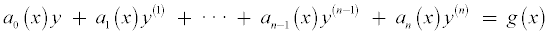is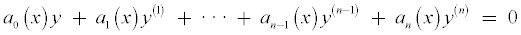Theorem 4.1. Let y1, y2, · · · , yn be linearly independent solutions of the homogeneous linear nth order differential equation(2.3)

on an interval I. Then the general solution of the equation in 2.3 on the interval I is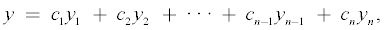where ci, (i=1, 2, · · · , n − 1, n) are arbitrary constants .

Theorem 4.2. Let y1, y2, · · · , yn be linearly independent solutions of the associated homogeneous linear nth order differential equation on an interval I, and let yp be particular solution of linear nth order differential equation

Then the general solution of the equation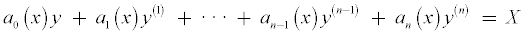on the interval I is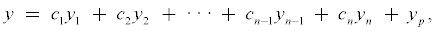where ci, (i = 1, 2, · · · , n − 1, n) are arbitrary constants .

General solution of linear second order differential equations: The second order linear ordinary differential equation with unknown dependent variable y and independent variable x is defined by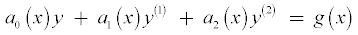(2.4)

Now, to solve nonhomogeneous second order linear differential equations, first we solve their associated homogeneous differential equations. Since the general solution of the equation in equation 2.4 consists of its particular solution, we search methods for solving the particular solution of equation in equation 2.4. Most authors of differential equations books used two famous methods, namely, undetermined coefficients methods and variation of parameters method to find particular solution of nonhomogeneous linear second order differential equations. Here we would like to introduce new method for finding particular solution of nonhomogeneous linear second order differential equations. Moreover, we construct a second solution of the associated homogeneous linear second order differential equation so that the set consisting of y1and y2 is linearly independent on I from this new method.

Procedure for deriving new method for particular solution of nonhomogeneous linear second order differential equations: Let y1 be a non-zero known solution of the associated homogeneous linear second order differential equation of the equation in equation 2.4. Thus, we have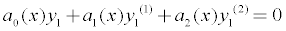(2.5)

Then we assume that yp(x) = u(x)y1(x) as particular solution of the equation in equation 2.4 to construct particular solution of the equation in equation 2.4.

It follows that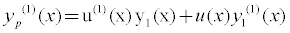and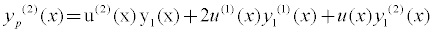Since yp(x)=u(x)y1(x) is particular solution of the equation in equation

2.4, we have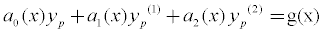(2.6)

From equation in equation 2.6 It follows that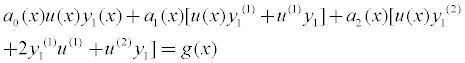(2.7)

Using equation in equation 2.5, the equation in equation 2.7 is reduced to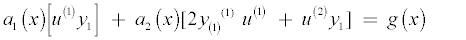(2.8)

From equation in equation 2.8 It follows that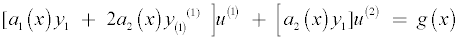(2.9)

Let u(1)=V. Then from equation in equation 2.9, we have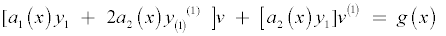(2.10)

The equation in equation 2.10 is linear first order ordinary differential equation. Thus,

using the formula in equation 2.2, we get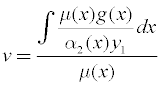(2.11)

where μ(x) =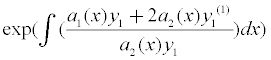Thus, u =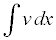, , whereand μ(x) =Clearly,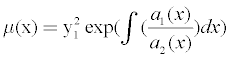Therefore, articular solution yp(x) = u(x) y1(x) of the equation in equation 2.4 is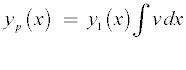(2.2)

whereand μ(x) =#### Result and Discussion

There are two particular solution methods. One is method of undetermined coefficients. The general method of undetermined coefficients is limited to differential equations of the form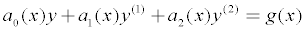, where a0(x) , a1(x) and a2(x) are constant functions and g(x) is a constant k, a polynomial function, an exponential function, a sine or cosine function, or finite sums and product of these functions . Thus, this method is not general method for particular solution. The second particular solution method is variation of parameters method. Unlike method of undetermined coefficients, this method is general method for particular solution because it works even if the conditions given for method of undetermined coefficients fail.

Now we derived the third method for particular solution ofas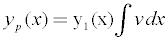, whereand y = c1y1 + c2y2 + yp ,

Unlike variation of parameters method, we need only one solution of the associated homogeneous differential equation of a differential equation to derive particular solution of a differential equation. Moreover, this new method for particular solution is good method because it works even if the conditions given for method of undetermined coefficients fail.

#### Conclusion

Now, we use equations in equation 2.12 to draw conclusion about particular solution method of the equation in equation 2.4 if y1(x) is known solution of the associated homogeneous equation of the equation in equation 2.4. Therefore, the particular solution method of the equation in equation 2.4 is,

Whereand μ(x) =From this particular solution method, one can construct the second solution of the associated homogeneous equation of the equation in equation 2.4 inserting g(x)=0 in this particular solution method. This implies that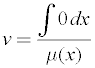. Thus choose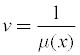Therefore,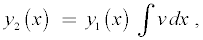Whereandis the second solution of the associated homogeneous equation of the equation in equation 2.4. Moreover, the general solution of the equation in equation 2.4 is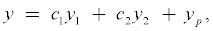where y1, y2 and yp are as given above. Here ci, (i=1, 2) are arbitrary constants.

#### References

Select your language of interest to view the total content in your interested language

### Article Usage

• Total views: 34524
• [From(publication date):
April-2015 - Nov 20, 2019]
• Breakdown by view type
• HTML page views : 30192Can't read the image? click here to refresh